# RSA Encryption

Alice and Bob have been friends forever… but Alice wants to tell Bob she loves him using a secret message (to prevent people from finding out and gossiping). Alice tells Bob she needs to give him a secret message and they decide to use the RSA algorithm. This is a type of public key cryptosystem, where anyone (like Alice) can encrypt their message but only one person (Bob) can decrypt it. Check out the visual I made below to see how Alice shares her secret to Bob 👀

Bob has successfully decrypted the message! There’s no way a public attacker, like Carl, can decrypt the message because he doesn’t know the value of the private prime numbers p and q, which he needs to find Ψn and then e. The only way he could find p and q is by prime factoring n. Luckily for Alice and Bob, no existing computers have the power to find p and q in a practical way. It would take thousands of years! By then, both Alice and Bob (and Carl) would be dead.

# Shor’s Algorithm

Enter quantum computers!

Quantum computers speed up the process of factorization by turning it into a period finding problem (more on that later). Shor’s algorithm harnesses these computers’ constructive/destructive interference and ability to make simultaneous calculations to cleverly get to the correct answer.

In fact, the “big O” (aka, number of computational steps needed to find the solution) for the Shor’s algorithm is exponentially less than that of a classical algorithm.

The algorithm has a classical part and a quantum part, which will be discussed below:

Classical Part

Here is a snippet of my code for a simple classical way to factorize. It can factorize the number N, which is 15.

How this works:

1. First it chooses a number a that is coprime with N. In other words, a and N do not share any common factors.
2. Then, it uses modular exponentiation to store 13^z mod 15 in a list for z ranging from 0 to 14. The mod function is basically the remainder you get when dividing 13^z with 15. For example, 13² mod 15 is 4. What is interesting is that these values end up repeating no matter how large 13^z gets. In the graph above, you can see that the y-axis repeats 0, 13, 4, and 7. Now that we have a repeating function, we need to find how often it repeats. This is denoted by the value “r”, called the order or period. r is the smallest number such that 13^r mod 15= 1.
3. Lastly, it checks if r is an even number. In this case, it assigns a variable x = 13^(r/2) (mod 15) and finds the prime numbers p and q by finding the greatest common factor between x+1 and N and x-1 and N. If r happens to be odd, we need to choose another number a that is coprime with N.

This sort of works but there’s a catch. If r is too large, it might be an issue because classical computers aren’t too great at calculating large exponents. It also gets tough to find the period of a more complicated function, which you see for larger values of N.

Where classical computers struggle with period finding (step 2) for such large numbers, quantum computers excel. They use calculations like the quantum Fourier Transform and Phase Estimation (insert cool sci-fi music) to accomplish this.

Quantum Part (aka quantum phase estimation)

Let’s say we were factoring the number 15 using a quantum computer.

How this works (note, the steps refer to the left):

1. Create two sets of four zero qubits. We are using four qubits because it can be used to represent 2⁴ (or 16) states, sufficient for N=15, which we are trying to factor.
2. Next, we add a Hadamard gate to the qubits on the x register (top). This effectively transforms the qubits from the computational basis to the Fourier basis.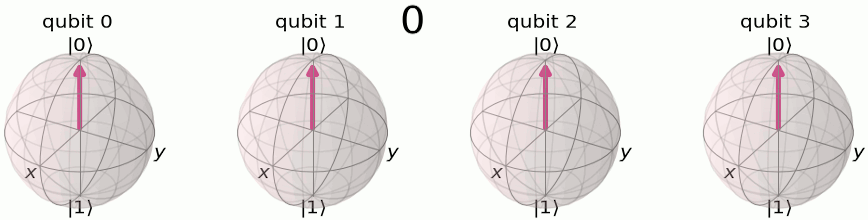These are qubits in the computational basis (aka, the z axis). Image from Qiskit Textbook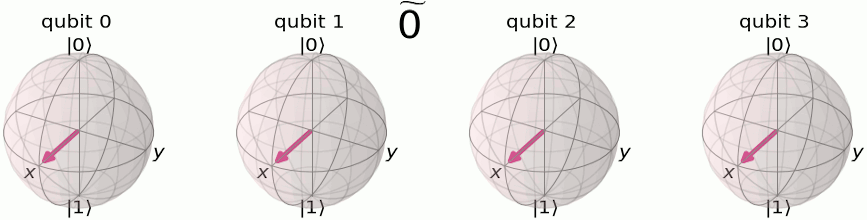The Hadamard gate transforms them to the Fourier basis (aka, the xy plane). Information is stored in terms of angles around the z-axis. Image from Qiskit Textbook

The x register now contains values from 0 to 15.

3. Now, we add a unitary operator, U, which uses modular exponentiation to bucket the values 0–15 from the x register into the values 1, 13, 4, and 7. Sounding familiar? That’s because this is analogous to step 2 of the classical part! The main difference is that quantum computers perform the modular exponentiation to all of the vectors 0–15 simultaneously, holding the values in a superposition. Here’s a mathematical representation of this step: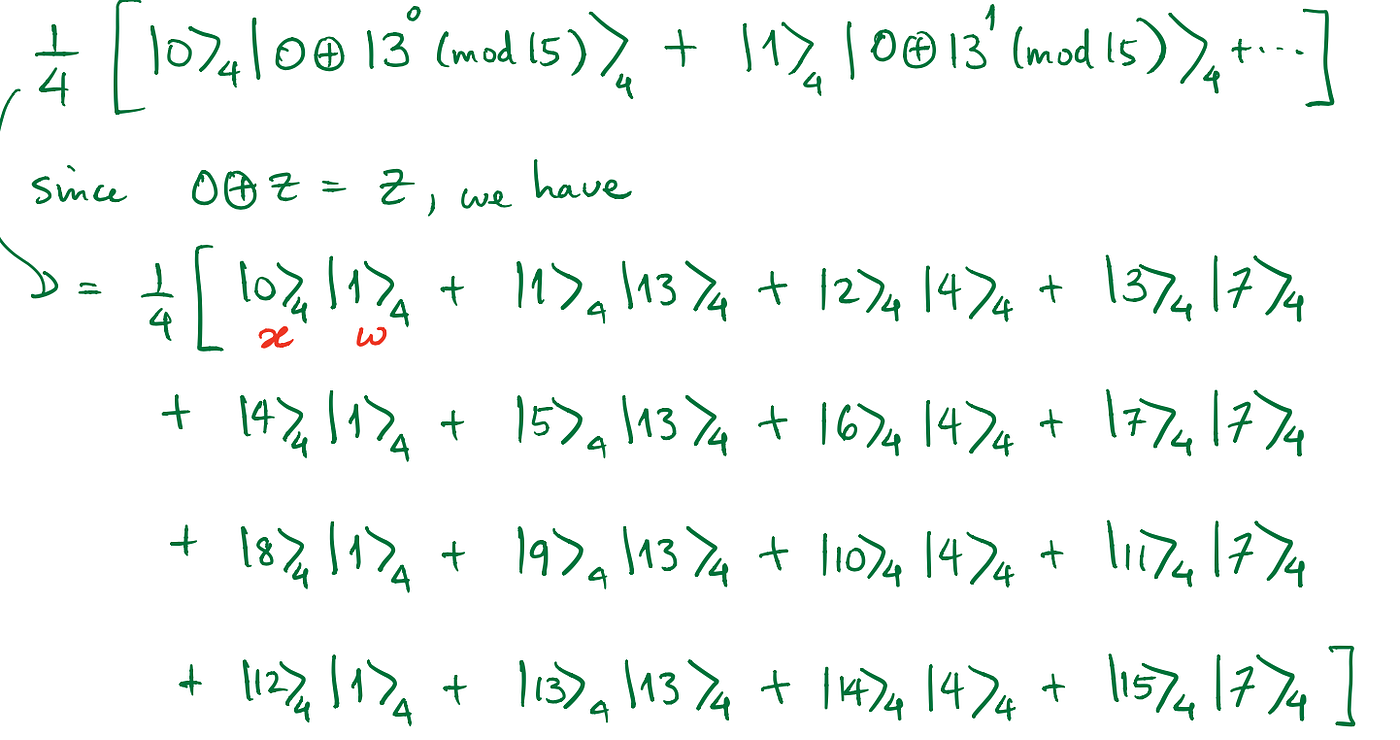Note: the left vector represents the x register and the right is the w register (which is in superposition to x). 1/4 is a normalization constant, and the plus sign with the circle around it is a bitwise operation. Image from Qiskit

3. This step is unnecessary, but lets do it for the sake of learning! Here’s what it would look like if we measured 7 on the w-register:

4. This is where we apply the QFT dagger (inverse quantum Fourier transform) to the qubits on the x-register. In other words, we’re taking these qubits out of the Fourier basis and back into the computational basis (recall step 2). During QFT dagger, most of the terms would cancel to zero (thanks to destructive interference) and you’d be left with 0, 4, 8, or 12 (the values where 13^r mod 15=1 would be 1!).

5. Now we measure the x-register. You’d have an equal chance of it collapsing into an answer of 0, 4, 8, or 12. With all of these different possible values, how can you find the factors of 15? Time for some classical post processing!

Classical Post-Processing

We’re at the final stretch! Now, we just need to analyze the measured value. We know these measurement outcomes are equal to some j(N/r) where j is an integer, N is 2⁴=16, and r is the period we’re looking for. After a little trial and error, we would plug r into step 3 of the first classical part.

• If we measure 0, we would have to run this again since this is a trivial solution (j would need to be 0 and r could be anything, so that doesn’t help us).
• If we measure 4, one pair of j and r is j=1 and r=4. You would run r=4 in step 3 and successfully find 3 and 5 as the factors.
• If we measure 8, one pair could be j=1 and r=2. Plugging in r=2 into step 3 yields the factors 1 and 3 which is a partial solution. In this case, we could just divide 15 by 3 to obtain the other factor, 5. If we were to plug in r=4 (for j=2) then we would get 3 and 5.
• If we measure 12, j=3 and r=4. We would get the factors 3 and 5.

And we’re done! Woohoo!! 🎉

# Pitfalls

As amazing as this Shor’s algorithm is, there are some issues.

• We don’t have enough qubits! The amount of qubits, n, we need is over log₂(N). In most general cases, we actually need 2n qubits. For example, a 1000 digit number we are trying to factorize will require 2000 qubits. Today’s quantum computers are not up to this level, so for now, RSA encryption is safe.
• It may not meet the conditions! There’s a chance (less than 50%) that r is not even or a^(r/2)+1=0. In this case, we will not be able to carry out the classical post-processing and find the prime factors.

# Conclusion

There are many steps and calculations involved to completely understand how the Shor’s algorithm works. Boiling it down, this algorithm uses quantum computers to turn factorization into a period finding problem and help find the period. Then it gives the period to classical computers to factorize. Luckily, Qiskit Aqua has created a module called Shor, allowing you to do this entire process with one line of code.

And now, the grand question: what does this mean for RSA encryption and the security of basically all of our online transactions? Well, to be honest, cybersecurity experts are trembling in fear of all of the Carls out there with eventually capable quantum computers. To prepare for post-quantum cryptography, experts are working on quantum-safe cryptography systems.

Beyond all of the math and equations, there are real electrons and photons using their mysterious quantum properties to carry out this incredibly impactful calculation. And that, I believe, is Shor to blow your mind.

--

--

--

## More from Aashvi Manakiwala

CS @ UPenn | TKS Activator | AI Enthusiast

Love podcasts or audiobooks? Learn on the go with our new app.

## Mathematics of kolam: folkloric graph theory## Saving the Japanese From Evil Mathematicians## Bellman-Ford Algorithm & its applications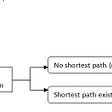## The Fun Side of Mathematics: Theorema Egregium## How To Read Books, But Only At Random## How Quantum Mechanics Alters Reality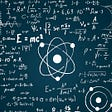## “Constant Curvature”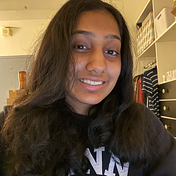## Aashvi Manakiwala

CS @ UPenn | TKS Activator | AI Enthusiast

## Securing Communication with Quantum Cryptography## Quantum Computer simulator from scratch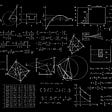## How to Host Your Own Quantum Computing Hackathon: A Guide